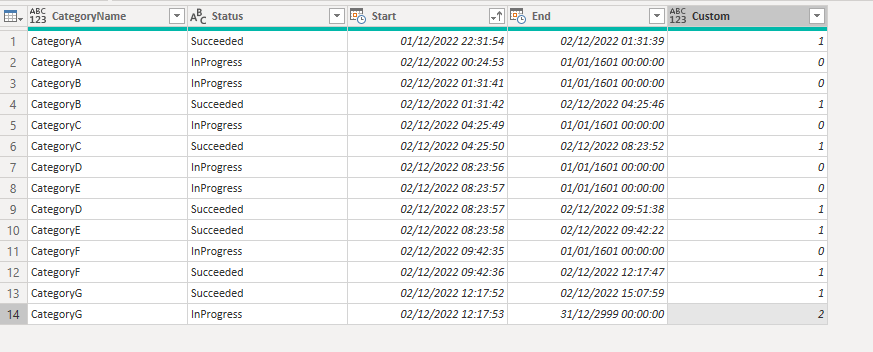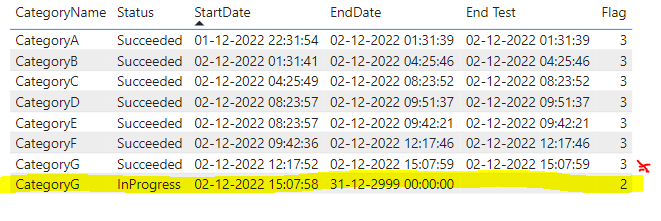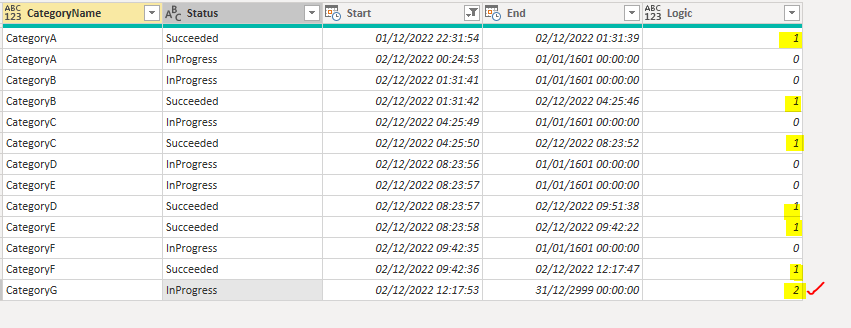cancel
Showing results for
Did you mean:Frequent Visitor

## Get the max of a value based on a category and date

Hi,

I've a table that shows me the category by state and the start and end date:and I'm trying to create a conditional column that returns the maximum of each category and status.

I tried to create the column in M

``Logic= if [Status] = "InProgress" and [Start] = List.Max(#"Sorted Rows1"[Start]) and [End] = #datetime(2999, 12, 31, 0, 0, 0) then 2 else if [Status] = "Succeeded" then 1 else 0``

​​and it is working correctly except for the case of CategoryG which should only return the value of "2" from the Logic column, and instead it is returning the maximum of the Succeeded status and the InProgress status, where it should only show the InProgress status because it has a longer start date.I also tried creating in DAX but got the same result (the StartDate is selected as "Earliest" and the EndDate and End Test as "Latest"):``````Flag DAX = SWITCH( TRUE (),
'Table'[Start]= MAX ( 'Table'[Start] )
&& 'Table'[End Test] = BLANK ()
&& 'Table'[Status] = "InProgress", 2,
'Table'[Status] = "Failed", 1,
'Table'[Start]= LASTNONBLANK(  'Table'[Start],1 )
&& 'Table'[Status] = "Succeeded"  && 'Table'[End Test] <> BLANK (), 3,
0
)``````

Desired output:Any idea how to solve this ?

Thanks and kind regards.

1 ACCEPTED SOLUTIONCommunity Support

Hi  @coding ,

You can try this measure:

``````Flag DAX = SWITCH( TRUE (),
MAX('Table'[Start])= CALCULATE(MAX('Table'[Start]),ALL('Table'))
&& MAX('Table'[End Test]) = BLANK ()
&& MAX('Table'[Status]) = "InProgress", 2,
MAX('Table'[Status]) = "Failed", 1,
MAX('Table'[Start])=CALCULATE(LASTNONBLANK('Table'[Start],1),ALL('Table'))
&& MAX('Table'[Status]) = "Succeeded"  && MAX('Table'[End Test]) <> BLANK (), 3,
0
)``````

If that's not what you need, provide sample files.

Best Regards,
Gallen Luo
If this post helps, then please consider Accept it as the solution to help the other members find it more quickly.

2 REPLIES 2Community Support

Hi  @coding ,

You can try this measure:

``````Flag DAX = SWITCH( TRUE (),
MAX('Table'[Start])= CALCULATE(MAX('Table'[Start]),ALL('Table'))
&& MAX('Table'[End Test]) = BLANK ()
&& MAX('Table'[Status]) = "InProgress", 2,
MAX('Table'[Status]) = "Failed", 1,
MAX('Table'[Start])=CALCULATE(LASTNONBLANK('Table'[Start],1),ALL('Table'))
&& MAX('Table'[Status]) = "Succeeded"  && MAX('Table'[End Test]) <> BLANK (), 3,
0
)``````

If that's not what you need, provide sample files.

Best Regards,
Gallen Luo
If this post helps, then please consider Accept it as the solution to help the other members find it more quickly.Super User

https://community.powerbi.com/t5/Community-Blog/How-to-provide-sample-data-in-the-Power-BI-Forum/ba-...Announcements#### Exclusive opportunity for Women!

Join us for a free, hands-on Microsoft workshop led by women trainers for women where you will learn how to build a Dashboard in a Day!#### Power Platform Conference-Power BI and Fabric Sessions

Join us Oct 1 - 6 in Las Vegas for the Microsoft Power Platform Conference.Top Solution Authors
Top Kudoed Authors
Users online (1,863)